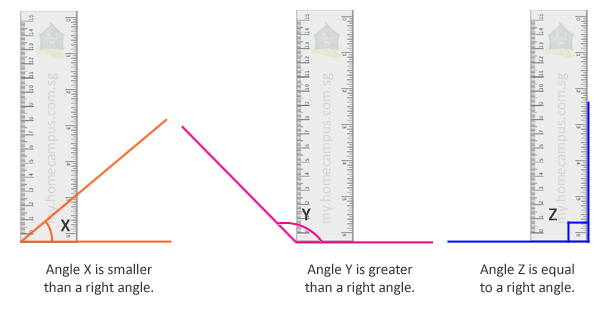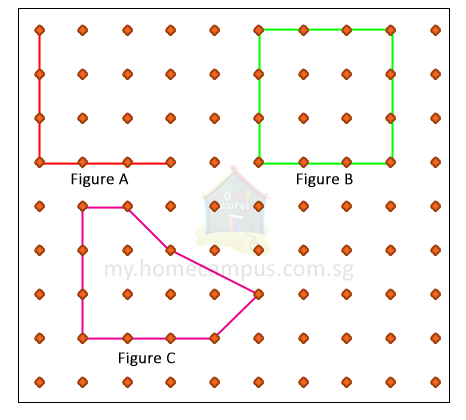## Right Angles

Practice Unlimited Questions

#### What is a right angle?

When an angle between two lines is exactly 90° it is called a right angle. Two lines are perpendicular to each other.

#### 1. Study the marked angles on the objects below.Each of the marked angles is a right angle.

#### 2. Which of the angles below is smaller than a right angle? Which one is greater than a right angle? Which one is a right angle?Let's use a ruler to check for right angles.

#### 3. How many right angles are there in each figure below?Figure A:Figure A has 1 right angle.

Figure B:Figure B has 4 right angles.

Figure C:Figure C has 2 right angles.